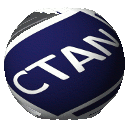Directory graphics/pgf/contrib/matrix-skeleton

matrix.skeleton is a PGF/TikZ library that simplifies working with multiple matrix nodes. To do so, matrix.skeleton correctly aligns groups of nodes with the content of the whole matrix. Furthermore, matrix.skeleton provides rows and columns for easy styling.

For example, the following picture is easily typeset:with this simple code:

\documentclass[tikz]{standalone}

\usetikzlibrary{matrix.skeleton}

\begin{document}
\begin{tikzpicture}
\matrix (m) [matrix of math nodes,
style odd rows on layer={background}{fill=black!25}] {
1  & \frac{\frac{16}{2}}{4}  & 3  \\
4.0000001  & 5  & 6  \\
7  & 8  & 9  \\
10 & 11 & 12 \\
13 & 14 & 15 \\
};
\fitandstyle{(m-cell-1-1) (m-cell-2-2)}{draw=red, fill=red!25, opacity=0.5}
\end{tikzpicture}
\end{document}